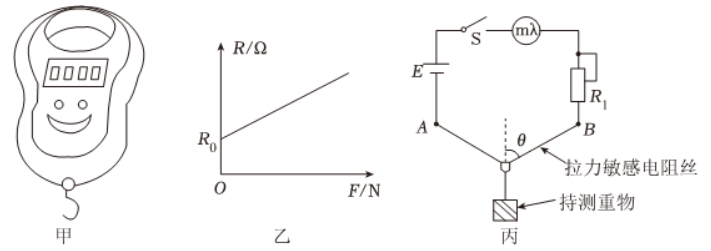(1) 试写出敏感电阻丝上的拉力 $F$ 与重物重力 $G$ 的关系式 $F=$
(2)设 $R-F$ 图像斜率为 $k$, 试写出电流表示数 $I$ 与待测重物重力 $G$ 的表达式 $I=$ ( 用 $B 、 r 、 R_1 、 R_k 、 R_0 、 k 、 \theta$ 表示)；
(3) 若 $R-F$ 图像中 $R_0=100 \Omega, k=0.5 \Omega / N$, 测得 $\theta=60^{\circ}$, 毫安表指针半偏, 则待测重物重力 $G=$ $N$ i
(4)若电源电动势不变, 内阻变大, 其他条件不变, 用这台“简易吊秤”, 称重前, 进行了步骤 $a$ 操作, 则测量结果 ( 填“偏 大”、“偏小”或“不变”）。